Courses

# NEET Previous Year Questions (Level 2): Waves

## 13 Questions MCQ Test Physics For JEE | NEET Previous Year Questions (Level 2): Waves

Description
This mock test of NEET Previous Year Questions (Level 2): Waves for JEE helps you for every JEE entrance exam. This contains 13 Multiple Choice Questions for JEE NEET Previous Year Questions (Level 2): Waves (mcq) to study with solutions a complete question bank. The solved questions answers in this NEET Previous Year Questions (Level 2): Waves quiz give you a good mix of easy questions and tough questions. JEE students definitely take this NEET Previous Year Questions (Level 2): Waves exercise for a better result in the exam. You can find other NEET Previous Year Questions (Level 2): Waves extra questions, long questions & short questions for JEE on EduRev as well by searching above.
QUESTION: 1

### In the ideal souble-slit experiment, when a glass - plate (refractive index 1.5) of thickness t is introduced in the path of one of the interfering beams (wavelength l), the intensity at the position where the central maximum occurred previously remains unchanged. The minimum thickness of the glass-plote is :   [JEE 2002]

Solution:

For minimum thickness, (n−1)t=λ
t= λ /(n−1) ​= λ /(1.5−1) ​=2λ

QUESTION: 2

### In the adjacent diagram, CP represents a wavefront and AO and BP, the corresponding two rays. Find the condition on q for constructive interference at P between the ray BP and reflected ray OP.     [JEE (Scr)2003]

Solution:

From geometry,
OP=d/cosθ
Path difference between the rays=CO+OP=d/cosθ cos2θ+d/cosθ​=2dcosθ
Since there is a phase change by π due to reflection from optically denser medium, condition for constructive interference is
Path difference=λ/2​
⟹cosθ=λ/4d​

QUESTION: 3

### A prism (mP =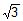) has an angle of prism A = 30°. A thin film (mf = 2.2) is coated on face AC as shown in the figure. Light of wavelength 550 nm is incident on the face AB at 60° angle of incidence. Find (i) the angle of its emergence from the face AC and (ii) the minimum thickness (in nm) of the film for which the emerging light is of maximum possible intensity.

Solution:
QUESTION: 4

In a YDSE bi-chromatic light of wavelengths 400 nm and 560 nm are used. The distance between the slits is 0.1 nm and the distance between the plane of the slits and the screen is 1m. The minimum distance between two successive regions of complete darkness is -

[JEE' 2004 (Scr)]

Solution:

Let nth minima of 400 nm wave coincides with mth minima of 560 nm wave.

Then,

(2n-1)(400/2) - (2m-1)(560/2)

2n-1 /2m-1  = 7/5 =14/10 ....
That is, 4th minima of 400 nm wave coincides with 3rd minima of 560 nm wave.
Location of this minima is-
y1 =[ (2×4-1).(1000).(400×10-6 ) ] / 2×0.1           (given, d=0.1 )
= 14 mm
Next 11th minima of 400 nm wave will coincide with 8thminima of 560 nm wave.
Location of this second minima is-
y2 = [(2×11-1).(1000).(400×10-6 )] /2×0.1
= 42 mm
So, the distance  between two successive regions of complete darkness is,
y2 - y1 = 42-14 = 28 mm

*Answer can only contain numeric values
QUESTION: 5

In a Young's double slit experiment, two wavelengths of 500 nm and 700 nm were used. What is the minimum distance from the central maximum where their maximas coincide again ? Take D/d = 103. Symbols have their usual meanings.

[JEE 2004]

Solution:

Now, mth max of 500nm coincides with nth of 700nm wavelength one.
⇒ ym = yn
⇒ nλ1 = mλ2
⇒ n x 700 = m x 500
⇒ n = 5 and m = 7 (minimum values)
⇒ Distance = yn = nλ1 D/d = 3.5 mm

QUESTION: 6

In Young's double slit experiment maximum intensity is I than the angular position where the intensity becomes 1/4 is -

[JEE' 2005 (Scr)]

Solution: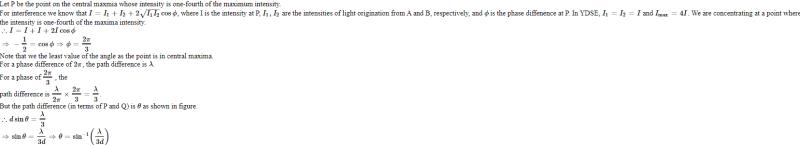QUESTION: 7

The figure shows a surface XY separating two transparent media, medium-1 and medium-2. The lines ab and cd represent wavefronts of a light wave traveling in medium-1 and incident on XY. The lines ef and gh represent wavefronts of the light wave in medium-2 after refraction.

[JEE 2007]

Light travels as a -

Solution:
QUESTION: 8

The phases of the light wave at c, d, e and f are fc, fd, fe and ff respectively. It is given that fc ¹ ff .

[JEE 2007]

Solution:

All points on a wave front are at the same phase.
∴ϕd​=ϕc​ and ϕf​=ϕe​
Subtracting both equations given above we get
ϕd​−ϕf​=ϕc​−ϕe

QUESTION: 9

Speed of light is

[JEE 2007]

Solution:
*Multiple options can be correct
QUESTION: 10

In a Young's double slit experiment, the separation between the two slits is d and the wavelength of the light is l. The intensity of light falling on slit 1 is four times the intensity of light falling on slit 2. Choose the correct choice(s).

[JEE 2008]

Solution:
QUESTION: 11

Column I shows four situations of standard Young's double slit arrangement with the screen placed far away from the slits S1 and S2 . In each of these cases S1P0 = S2 P0 , S1 P1 _ S2 P1 = l / 4 and S1P2 _ S2 P2 = l/3, where l is the wavelength of the light used. In the casesB,and D, a transparent sheet of refractive index m and thickness is pasted on slit S2 . The thicknesses of the sheets are different in different cases. The phase difference between the light waves reaching a point on the screen from the two slits is denoted by d(P) and the intensity by I(P). Match each situation given in Column I with the statement(s) in Column II valid for that situation. [JEE 2009]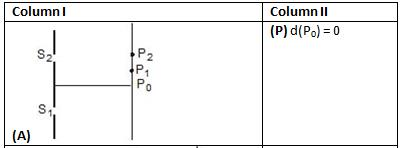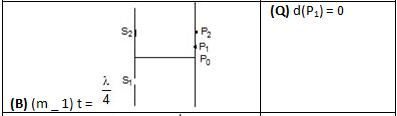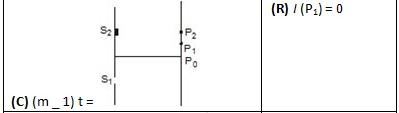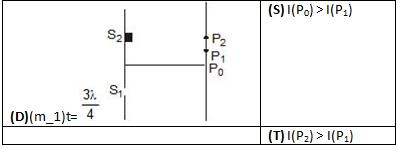Solution:
QUESTION: 12

Young's double slit experiment is carried out by using green, red and bluelight, one color at a time. The fringe widths recorded are bG, bR and bb, respectively. Then.

[JEE 20012]

Solution:

We know that,
fringe width, β=λD/d and hence
Now we also know that, β∝λ
λRGB
therefore,
βRGB

QUESTION: 13

In the young's double slit experiment using a monochromatic light of wavelength l, the path difference (in terms of an integer n) corresponding to any point having half the peak intensity is :

[JEE 2013]

Solution: